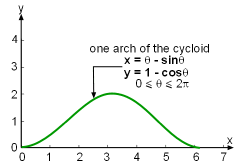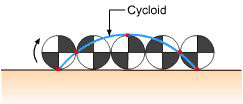Ch 10. Parametric Equations Multimedia Engineering Math ParametricEquations Tangentsand Areas Arc Length Surface Area
 Chapter 1. Limits 2. Derivatives I 3. Derivatives II 4. Mean Value 5. Curve Sketching 6. Integrals 7. Inverse Functions 8. Integration Tech. 9. Integrate App. 10. Parametric Eqs. 11. Polar Coord. 12. Series Appendix Basic Math Units Search eBooks Dynamics Fluids Math Mechanics Statics Thermodynamics Author(s): Hengzhong Wen Chean Chin Ngo Meirong Huang Kurt Gramoll ©Kurt GramollMATHEMATICS - CASE STUDY IntroductionProblem Diagram Matthew has just joined a computer company as a software engineer. One of the products that the company developed is an engineering drawing software. In engineering design, engineers often use a curve and revolve it around an axis to create a surface. Matthew's immediate task is to enhance this feature so that it also provides the users with the value of the surface area generated. How can Matthew find the surface area? QuestionsCycloid Determine the surface area generated from revolving a curve around an axis. Take one arch of the cycloid as an example. Approach Recall that a cycloid is a curve traced by rolling a circle along a flat surface, as shown in the animation. The parametric equations of the cycloid are given by: x = θ - sinθ and y = 1 - cosθ for 0 ≤ θ ≤ 2π.